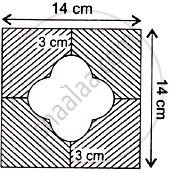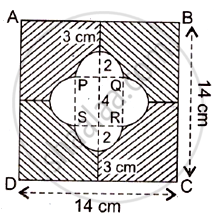# Find the Area of the Shaded Region Use π = 3.14 - Mathematics

Sum

In Figure, find the area of the shaded region [Use π = 3.14]#### Solution

From the symmetry of the given figure, we haveLet the radius of semicircle, r = d/2 = 4/2 = 2cm (Diameter of semicircle, d = 14/2 - 3 = 4 cm )

Let the side of small square = a = 4 cm (Side = Diameter of semicircle
Area of square ABCD = (Side)2 = (A)2 = (14)2 = 196cm2
Area of small square PQRS = (Side)2 = (a)2 = (4)2 = 16cm2

Area of 4 Semicircle = 4 x 1/2 pir^2

= 4 x 1/2 xx 3.14 xx 2^2 = 25.12 cm^2

Required area = ( Area of big square – Area of small square  – Area of 4 semicircles ) = ( 196 - 16 - 25.12 ) = 154.88 cm2

Concept: Circumference of a Circle
Is there an error in this question or solution?
2014-2015 (March) Delhi Set 1

Share